## Falling p.6

### Seeing quantum mechanical falling

Classical mechanics (and most people above age 3) have the notion of a particle's position. Quantum mechanics says that a particle doesn't have a position, and says it applies to all objects: big (where it replaces classical mechanics) and small (where classical mechanics wasn't working too well). Now unless we've all been living in a dream world since age 3, QM must have something that corresponds to our very real impression that objects have position. QM finds that "normal" objects under "normal" conditions have a range of possible positions that is so small, that for most purposes we can take the center of the range of possible positions as "the" position. For example if we wanted to localize a bacteria cell (1 µm) to an accuracy of 1/10 its diameter, the resulting uncertainty in speed would be: 10-12 m/s, for a Bucky Ball (C60): .04 m/s, while for a helium atom: 800 m/s (which is comparable to its normal speed at room temperature). Thus for most any object bigger than a small molecule, the range of possible positions required by quantum mechanics, is so small, that usually no harm results for just saying that the particle is in the middle of the QM range. (This statement is hedged because physicists work hard to make macroscopic systems in which quantum mechanics is important; but these rare beasts are rarely found outside of the lab.)

Now it turns out that if we use the information that the particle is approximately at a particular location, we must give up the idea that the particle has a definite energy. The resulting probability density then does depend on time: the middle of the probability density (which in the above we connect with the idea of "a" position) is seen to move much as classical mechanics says its mythical point particles would move.

Let's start by seeing how this would all work classically: we say the particle starts approximately at z0 but with some uncertainty: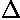z0. We release the particle on average with zero initial velocity, but because of Heisenberg uncertainty relation, there must be some spread of velocityv0, which is sometime up and sometimes down. If we knew exactly where the particle was starting from and its speed, we'd know exactly where it was:

z(t)=z0+v0tat2:

But because of the initial uncertainty in the velocity, there is growing uncertainty in position:z(t)= [(z0)2+ (v0t)2]½

Thus the packet of probability is becoming wider as the central value of the probability follows the usual classical relation:

z(t)=z0at2

In our dimensionless variables this reads:

z'(t')=z'0-t'2

Our particular application will have z'0=121.5,z'0=0.873,v'0=1.50.

Here is the behavior of the classical probability distributions:Here are the quantum mechanical results for t'=0,1,2,3,4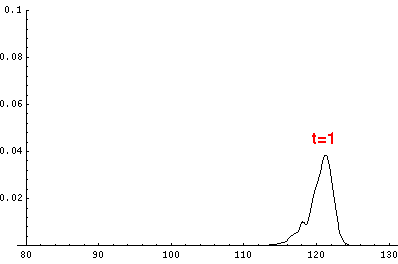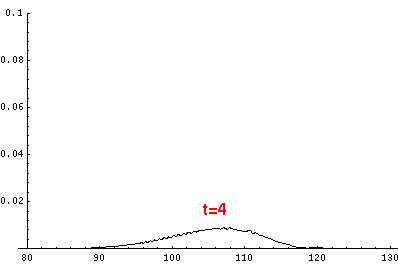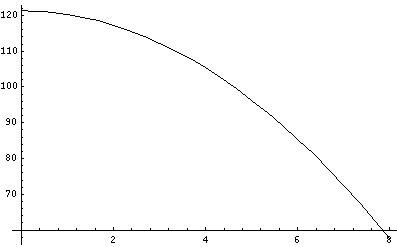The last plot shows the average position of the wavefunction vs time. All of the above look much like the classical result. Note that the "broadening" of the wavefunction is simply the result of our uncertainty in the initial velocity. Since the motion is periodic, one period later the particle must be back where it started, so the wavefunction "narrows" on the upward bounce, and looks like the below one period (t'=22) later: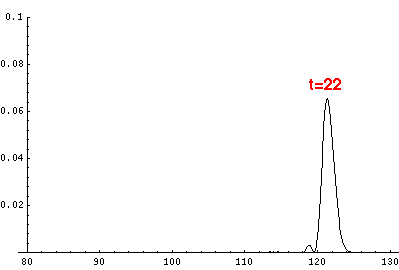For reasons that should be clear after the next page, over several periods the wave packet will broaden out and eventually the probability will be distributed throughout (and a bit beyond) the classically allowed region.

Here is some data on the location of the particle:

TimeHeight
0121.242
1120.245
2117.257
4105.309
685.411
857.603